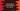# How to add elements to a Java vector using index# Add elements to a java vector using index :

Vector is a good replacement of array in Java if you want to add elements dynamically. We can add elements dynamically to a vector and it will increase its size, unlike arrays. Previously we have learned different examples of vectors like how to create vectors, how to add elements to a vector and how to clear a vector. In this tutorial, we will learn how to add elements to a vector in a specific position, i.e. using index numbers.

Following method we are going to use for adding new elements to a vector using the index :

``public void add(int index, E element)``

The method add takes two parameters: the first one is the index where we are adding the element and the second parameter is an element to be inserted.

This method will add the element at the specific index and moves all other elements to the right if available. Be careful to use the proper index while using this method. If the index is not valid, it will throw one exception. For example, if you are trying to add one element to the 2nd _index to an empty vector, it will throw _ArrayIndexOutOfBoundsException.

### Java Example :

``````import java.util.Vector;
public class Example {
public static void main(String[] args) {
Vector<string> strVector = new Vector<>();

//1
//2
System.out.println(strVector);

//3
//4
System.out.println(strVector);
}
}``````

### Output :

``````[one, two, three]
[one, four, two, three]``````

### Explanation :

The commented numbers in the above program denote the step numbers below :

1. Add three elements to the vector strVector. The elements are added to the 0,1 and 2 positions.
2. Print out the vector. It will print_ [one, two, three]._
3. Now add one more element ’four’ to the position_ ‘1’_ of the vector.
4. We already have element ’two’ on position ‘1’. So, all elements will move to the right and the new element will add to the first position. It will print_ [one, four, two, three]._

This program is shared on Github.

### Conclusion :

We have learned how to use add method to add elements to a vector in Java. This method comes in handy if you need to add an element to the middle of the vector. Try to run the example above and drop one comment below if you have any queries.